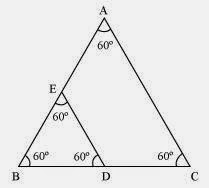Guru

# ABC and BDE are two equilateral triangles such that D is the mid-point of BC. Ratio of the area of triangles ABC and BDE is (A) 2 : 1 (B) 1 : 2 (C) 4 : 1 (D) 1 : 4 Q.8

• 0

The best way to solve the question of exercise 6.4 of triangles for class 10th what is the easiest way to solve this question ABC and BDE are two equilateral triangles such that D is the mid-point of BC. Ratio of the area of triangles ABC and BDE is (A) 2 : 1 (B) 1 : 2 (C) 4 : 1 (D) 1 : 4

Share

1. Given, ΔABC and ΔBDE are two equilateral triangle. D is the midpoint of BC.∴ BD = DC = 1/2BC

Let each side of triangle is 2a.

As, ΔABC ~ ΔBDE

∴ Area(ΔABC)/Area(ΔBDE) = AB2/BD2 = (2a)2/(a)2 = 4a2/a2 = 4/1 = 4:1

Hence, the correct answer is (C).

• 0